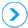Chit fund schemes possess a predetermined chit value and duration. The amount collected from members is auctioned out every month. Bidders can bid up to a maximum 40 % of this total collected value. The difference between the gross sum collected and the actual auction amount, known as the discount/bid amount, is then equally distributed among subscribers, or, is deducted from the next month’s premium.

### Let us take an example of a scheme of 40 members contributing Rs.5,000 each per month, for 40 months.

#### The total amount collected per month would be Rs. 20,00,00.

Suppose in the 1st month, 2 members X & Y need funds and participate in the bidding. Member X bids for Rs.75,000, Member Y bids for Rs.80,000 (40%). Thus, Member Y becomes eligible to draw the prize money for the month as his bid is higher than the first members bid.

Member Y can now withdraw

Rs.2,00,000 – Rs.80,000 (Discount/bid amount) Rs.1,500 (S/T @15% under revision) = Rs. 1,18,500/-.

Rs.80,000 – Rs.10,000 (Foreman commission of 5%) = Rs.70,000

70,000/40 = Rs.1,750 is distributed equally among all the members, ie: Rs.1,750 each.

So each subscriber will have to pay Rs.5000-Rs.1750/-(Dividend Amount) = Rs.3,250/-

So in effect, during the 3rd month, each member contributes only Rs.3,250 (Rs.5,000 (Monthly subscription) – Rs1,750 (Dividend).

This process is repeated for all 40 months till the end of the tenure, giving each member a chance of receiving the prize money. If in a particular month there are more than one person bidding for the same bid, a lottery is drawn to determine which of the member is eligible to withdraw the prize amount.